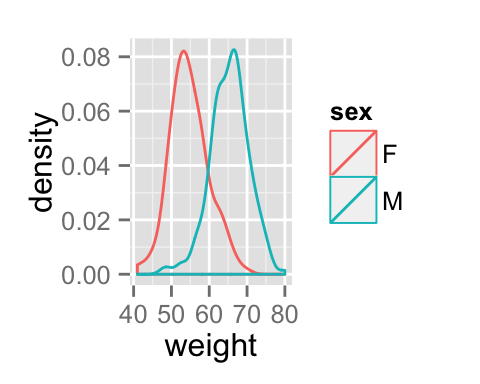# ggplot2 density plot : Quick start guide - R software and data visualization

This R tutorial describes how to create a density plot using R software and ggplot2 package.

The function geom_density() is used. You can also add a line for the mean using the function geom_vline.# Prepare the data

This data will be used for the examples below :

``````set.seed(1234)
df <- data.frame(
sex=factor(rep(c("F", "M"), each=200)),
weight=round(c(rnorm(200, mean=55, sd=5),
rnorm(200, mean=65, sd=5)))
)
``````##   sex weight
## 1   F     49
## 2   F     56
## 3   F     60
## 4   F     43
## 5   F     57
## 6   F     58``````

# Basic density plots

``````library(ggplot2)
# Basic density
p <- ggplot(df, aes(x=weight)) +
geom_density()
p
p+ geom_vline(aes(xintercept=mean(weight)),
color="blue", linetype="dashed", size=1)``````# Change density plot line types and colors

``````# Change line color and fill color
ggplot(df, aes(x=weight))+
geom_density(color="darkblue", fill="lightblue")
# Change line type
ggplot(df, aes(x=weight))+
geom_density(linetype="dashed")``````Read more on ggplot2 line types : ggplot2 line types

# Change density plot colors by groups

## Calculate the mean of each group :

``````library(plyr)
mu <- ddply(df, "sex", summarise, grp.mean=mean(weight))
``````##   sex grp.mean
## 1   F    54.70
## 2   M    65.36``````

## Change line colors

Density plot line colors can be automatically controlled by the levels of sex :

``````# Change density plot line colors by groups
ggplot(df, aes(x=weight, color=sex)) +
geom_density()
p<-ggplot(df, aes(x=weight, color=sex)) +
geom_density()+
geom_vline(data=mu, aes(xintercept=grp.mean, color=sex),
linetype="dashed")
p``````It is also possible to change manually density plot line colors using the functions :

• scale_color_manual() : to use custom colors
• scale_color_brewer() : to use color palettes from RColorBrewer package
• scale_color_grey() : to use grey color palettes
``````# Use custom color palettes
p+scale_color_manual(values=c("#999999", "#E69F00", "#56B4E9"))
# Use brewer color palettes
p+scale_color_brewer(palette="Dark2")
# Use grey scale
p + scale_color_grey() + theme_classic()``````Read more on ggplot2 colors here : ggplot2 colors

## Change fill colors

Density plot fill colors can be automatically controlled by the levels of sex :

``````# Change density plot fill colors by groups
ggplot(df, aes(x=weight, fill=sex)) +
geom_density()
# Use semi-transparent fill
p<-ggplot(df, aes(x=weight, fill=sex)) +
geom_density(alpha=0.4)
p
p+geom_vline(data=mu, aes(xintercept=grp.mean, color=sex),
linetype="dashed")``````It is also possible to change manually density plot fill colors using the functions :

• scale_fill_manual() : to use custom colors
• scale_fill_brewer() : to use color palettes from RColorBrewer package
• scale_fill_grey() : to use grey color palettes
``````# Use custom color palettes
p+scale_fill_manual(values=c("#999999", "#E69F00", "#56B4E9"))
# use brewer color palettes
p+scale_fill_brewer(palette="Dark2")
# Use grey scale
p + scale_fill_grey() + theme_classic()``````Read more on ggplot2 colors here : ggplot2 colors

# Change the legend position

``````p + theme(legend.position="top")
p + theme(legend.position="bottom")
p + theme(legend.position="none") # Remove legend``````The allowed values for the arguments legend.position are : “left”,“top”, “right”, “bottom”.

Read more on ggplot legends : ggplot2 legends

# Combine histogram and density plots

• The histogram is plotted with density instead of count values on y-axis
• Overlay with transparent density plot
``````# Histogram with density plot
ggplot(df, aes(x=weight)) +
geom_histogram(aes(y=..density..), colour="black", fill="white")+
geom_density(alpha=.2, fill="#FF6666")
# Color by groups
ggplot(df, aes(x=weight, color=sex, fill=sex)) +
geom_histogram(aes(y=..density..), alpha=0.5,
position="identity")+
geom_density(alpha=.2) ``````# Use facets

Split the plot in multiple panels :

``````p<-ggplot(df, aes(x=weight))+
geom_density()+facet_grid(sex ~ .)
p
p+geom_vline(data=mu, aes(xintercept=grp.mean, color="red"),
linetype="dashed")``````Read more on facets : ggplot2 facets

# Customized density plots

``````# Basic density
ggplot(df, aes(x=weight, fill=sex)) +
geom_density(fill="gray")+
geom_vline(aes(xintercept=mean(weight)), color="blue",
linetype="dashed")+
labs(title="Weight density curve",x="Weight(kg)", y = "Density")+
theme_classic()
# Change line colors by groups
p<- ggplot(df, aes(x=weight, color=sex)) +
geom_density()+
geom_vline(data=mu, aes(xintercept=grp.mean, color=sex),
linetype="dashed")+
labs(title="Weight density curve",x="Weight(kg)", y = "Density")

p + scale_color_manual(values=c("#999999", "#E69F00", "#56B4E9"))+
theme_classic()``````Change line colors manually :

``````# Continuous colors
p + scale_color_brewer(palette="Paired") + theme_classic()
# Discrete colors
p + scale_color_brewer(palette="Dark2") + theme_minimal()
p + scale_color_brewer(palette="Accent") + theme_minimal()``````Read more on ggplot2 colors here : ggplot2 colors

# Infos

This analysis has been performed using R software (ver. 3.1.2) and ggplot2 (ver. 1.0.0)